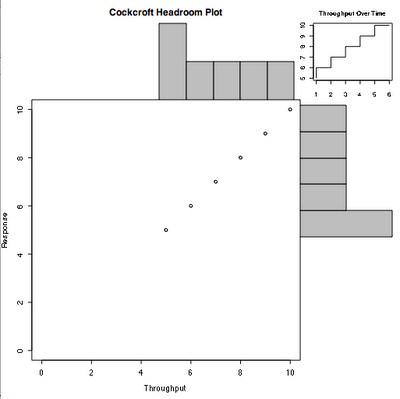Thursday, November 23, 2006

Cockcroft Headroom Plot - Part 3 - Histogram Fixes

I found that I had some scaling issues with the histograms that needed fixing. Ultimately this made the code look a lot more complex, but it now deals with scaling the plot and the histogram with a fixed zero origin on both axes. I think its important to maintain the zero origin for a throughput vs. response time plot.

The tricky part is that the main plot is automatically oversized from its data range by a few percent, and the units used in the histogram are completely different. A histogram with 6 bars is scaled to have the bars at unit intervals and is 6 wide plus the width of the bars etc. After lots of trial and error, I made the main plot use the maximum bucket size of the histogram as its max value, and artificially offset the histograms by what looks like about the right amount. The plot below uses fixed data as a test. You can see that the first bar includes two points, thats due to the particular algorithm used by R. Some alternative histogram algorithms are available, but this one seems to be most appropriate to throughput/response time data.

> chp(5:10,5:10)The updated code follows.

chp <- function(x,y,xl="Throughput",yl="Response",tl="Throughput Over Time",
xhist <- hist(x,plot=FALSE)
yhist <- hist(y, plot=FALSE)
xbf <- xhist\$breaks # first
ybf <- yhist\$breaks # first
xbl <- xhist\$breaks[length(xhist\$breaks)] # last
ybl <- yhist\$breaks[length(yhist\$breaks)] # last
xcl <- length(xhist\$counts) # count length
ycl <- length(yhist\$counts) # count length
xrange <- c(0,xbl)
yrange <- c(0,ybl)
nf <- layout(matrix(c(2,4,1,3),2,2,byrow=TRUE), c(3,1), c(1,3), TRUE)
layout.show(nf)
par(mar=c(5,4,0,0))
plot(x, y, xlim=xrange, ylim=yrange, xlab=xl, ylab=yl)
par(mar=c(0,4,3,0))
barplot(xhist\$counts, axes=FALSE,
xlim=c(xcl*0.03-xbf/((xbl-xbf)/(xcl-0.5)),xcl*0.97),
ylim=c(0, max(xhist\$counts)), space=0, main=ml)
par(mar=c(5,0,0,1))
barplot(yhist\$counts, axes=FALSE, xlim=c(0,max(yhist\$counts)),
ylim=c(ycl*0.03-ybf/((ybl-ybf)/(ycl-0.5)),ycl*0.97),
space=0, horiz=TRUE)
par(mar=c(2.5,1.7,3,1))
plot(x, main=tl, cex.axis=0.8, cex.main=0.8, type="S")
}